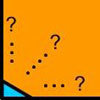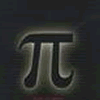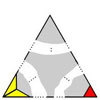# Resources tagged with: Rational and irrational numbers

Filter by: Content type:
Age range:
Challenge level:

### There are 20 results

Broad Topics > Numbers and the Number System > Rational and irrational numbers### Be Reasonable

##### Age 16 to 18 Challenge Level:

Prove that sqrt2, sqrt3 and sqrt5 cannot be terms of ANY arithmetic progression.### An Introduction to Proof by Contradiction

##### Age 14 to 18

An introduction to proof by contradiction, a powerful method of mathematical proof.### Irrational Arithmagons

##### Age 16 to 18 Challenge Level:

Can you work out the irrational numbers that belong in the circles to make the multiplication arithmagon correct?### Impossible Square?

##### Age 16 to 18 Challenge Level:

Can you make a square from these triangles?### Proof Sorter - the Square Root of 2 Is Irrational

##### Age 16 to 18 Challenge Level:

Try this interactivity to familiarise yourself with the proof that the square root of 2 is irrational. Sort the steps of the proof into the correct order.### An Introduction to Irrational Numbers

##### Age 14 to 18

Tim Rowland introduces irrational numbers### Good Approximations

##### Age 16 to 18 Challenge Level:

Solve quadratic equations and use continued fractions to find rational approximations to irrational numbers.### Approximations, Euclid's Algorithm & Continued Fractions

##### Age 16 to 18

This article sets some puzzles and describes how Euclid's algorithm and continued fractions are related.### Impossible Triangles?

##### Age 16 to 18 Challenge Level:

Which of these triangular jigsaws are impossible to finish?### Continued Fractions II

##### Age 16 to 18

In this article we show that every whole number can be written as a continued fraction of the form k/(1+k/(1+k/...)).### What Are Numbers?

##### Age 7 to 18

Ranging from kindergarten mathematics to the fringe of research this informal article paints the big picture of number in a non technical way suitable for primary teachers and older students.### Rational Round

##### Age 16 to 18 Challenge Level:

Show that there are infinitely many rational points on the unit circle and no rational points on the circle x^2+y^2=3.##### Age 16 to 18 Short Challenge Level:

Can you work out where the blue-and-red brick roads end?### Rational Roots

##### Age 16 to 18 Challenge Level:

Given that a, b and c are natural numbers show that if sqrt a+sqrt b is rational then it is a natural number. Extend this to 3 variables.### The Clue Is in the Question

##### Age 16 to 18 Challenge Level:

Starting with one of the mini-challenges, how many of the other mini-challenges will you invent for yourself?### Equal Equilateral Triangles

##### Age 14 to 16 Challenge Level:

Can you make a regular hexagon from yellow triangles the same size as a regular hexagon made from green triangles ?### The Square Hole

##### Age 14 to 16 Challenge Level:

If the yellow equilateral triangle is taken as the unit for area, what size is the hole ?### Rationals Between...

##### Age 14 to 16 Challenge Level:

What fractions can you find between the square roots of 65 and 67?### Repetitiously

##### Age 14 to 16 Challenge Level:

Can you express every recurring decimal as a fraction?### Spirostars

##### Age 16 to 18 Challenge Level:

A spiropath is a sequence of connected line segments end to end taking different directions. The same spiropath is iterated. When does it cycle and when does it go on indefinitely?